# RD Sharma Solutions for Class 11 Chapter 16 - Permutations Exercise 16.5

So far we have discussed permutations of distinct objects by taking some or all at time. In this section, we intend to discuss the permutations of a given number of objects, when objects are not all different. Important theorems are explained in simple steps to help students remember them without confusion. Students get a better idea about the concepts covered in this exercise by making use of RD Sharma Class 11 Maths Solutions pdf, which in turn, helps students boost their exam preparation. To make it easier for the students, free pdf is available here in downloadable format.

## Download the pdf of RD Sharma Solutions for Class 11 Maths Exercise 16.5 Chapter 16 – Permutations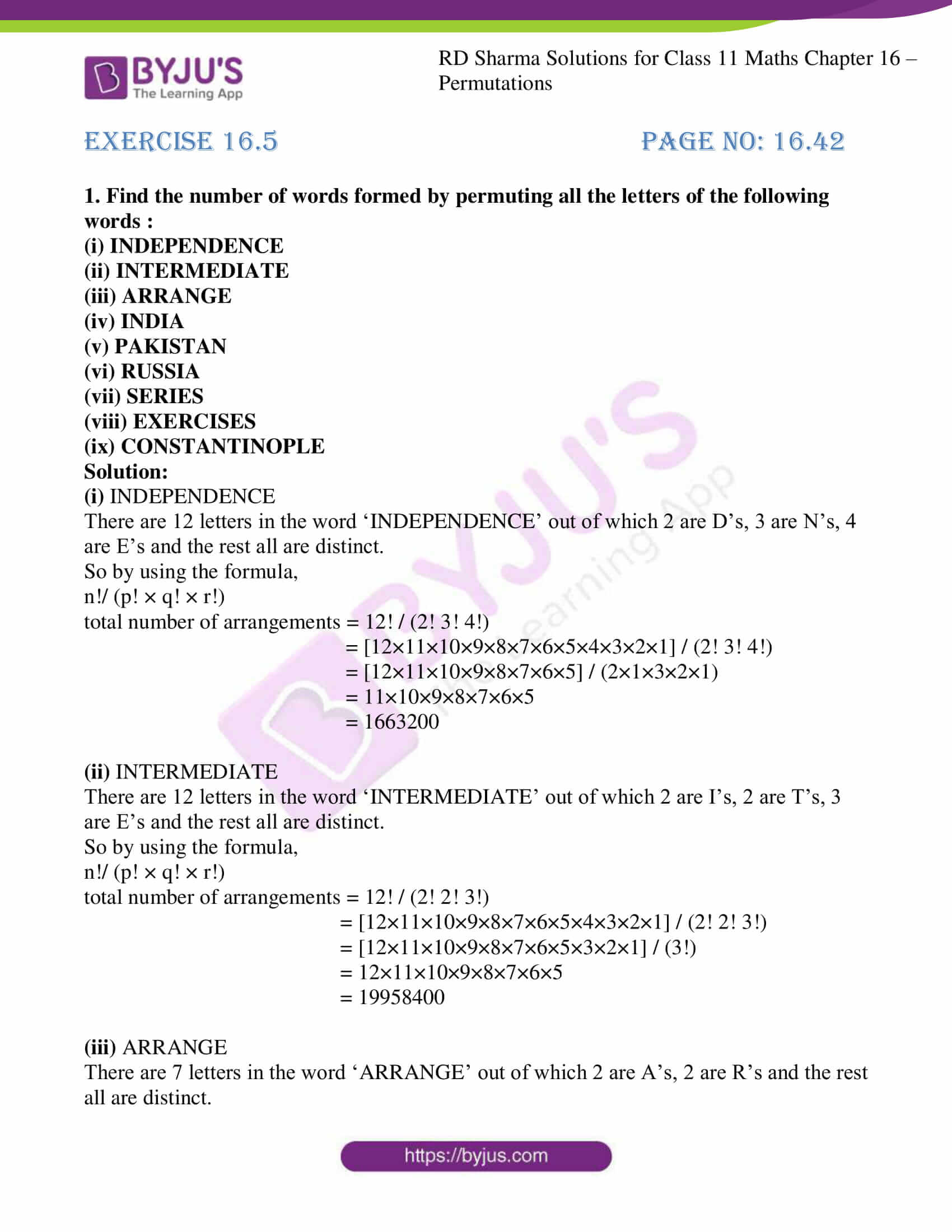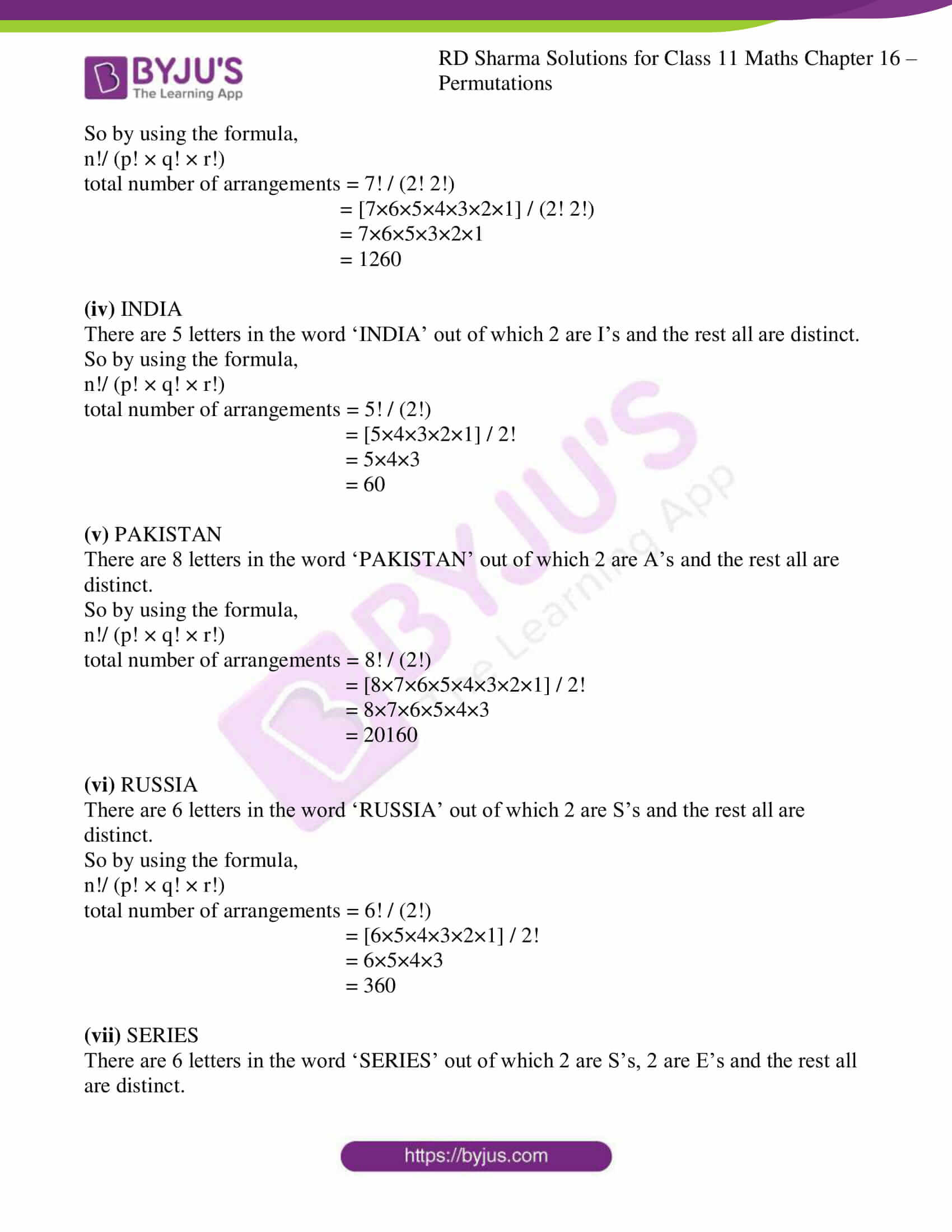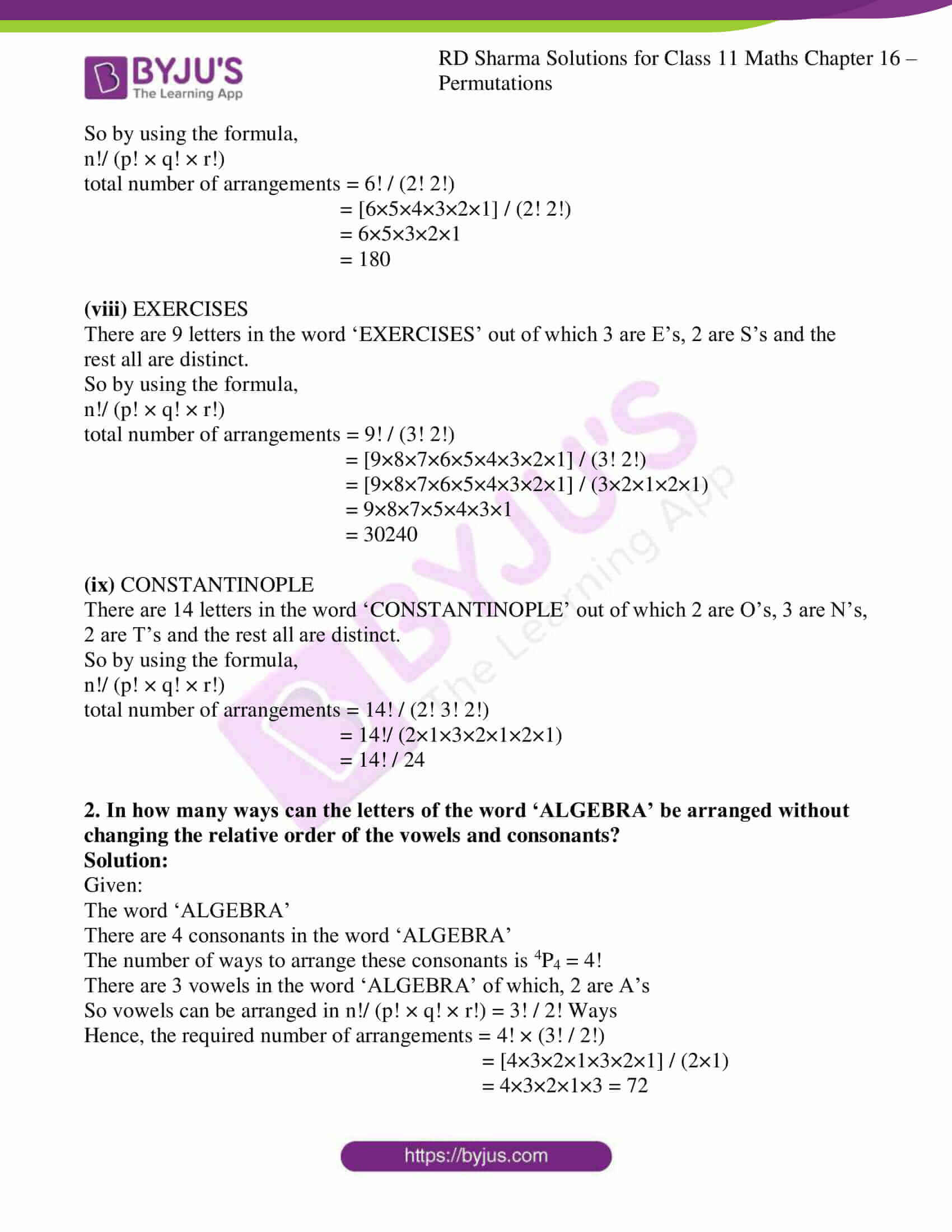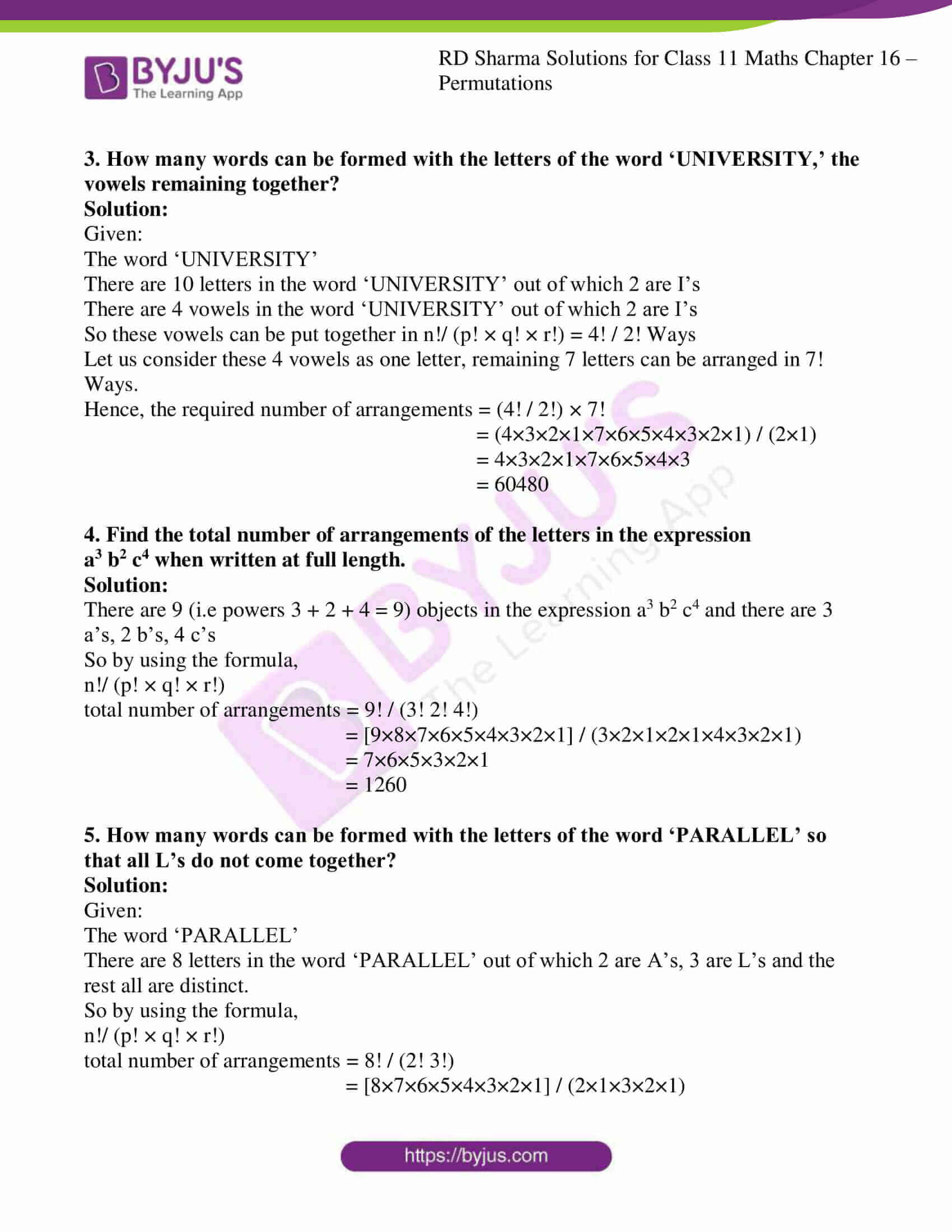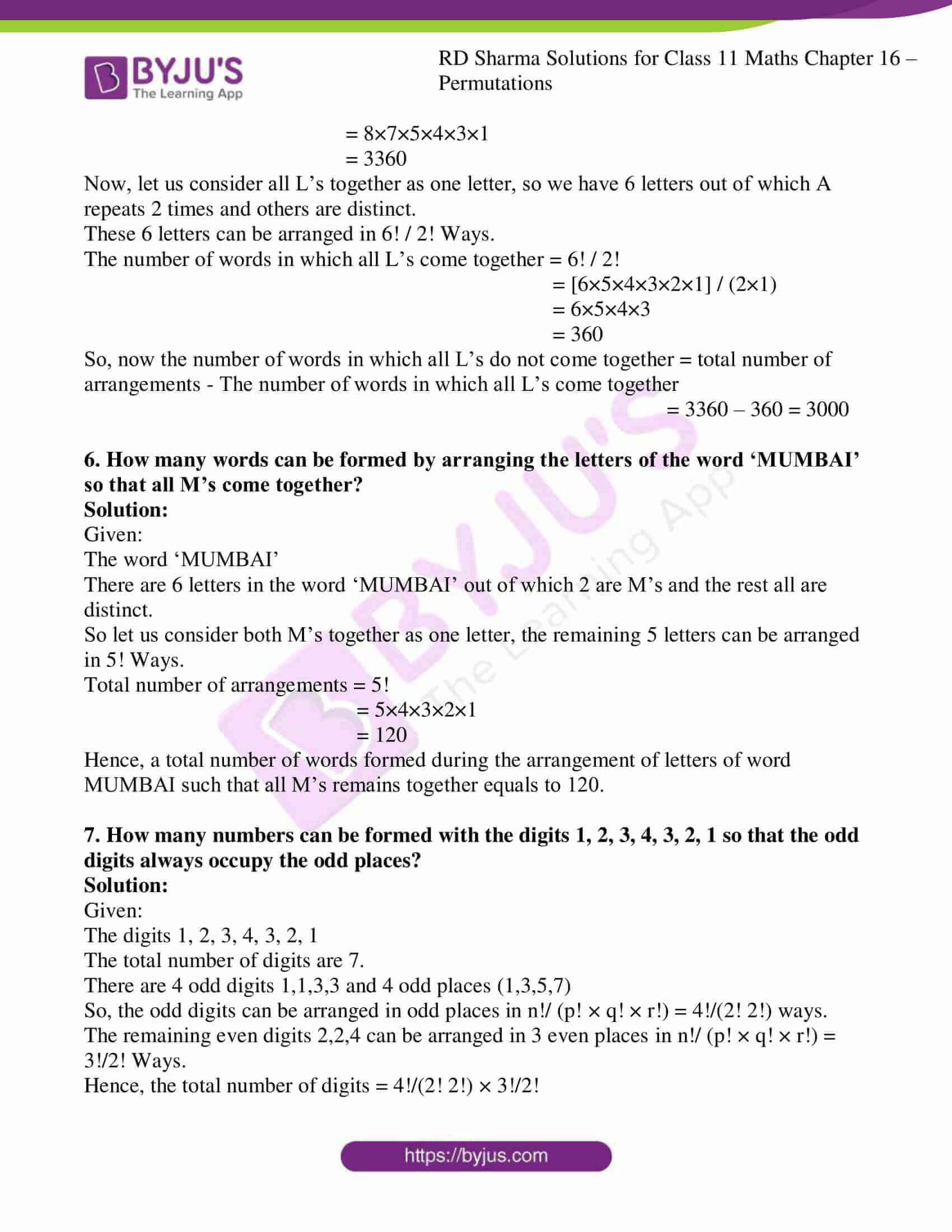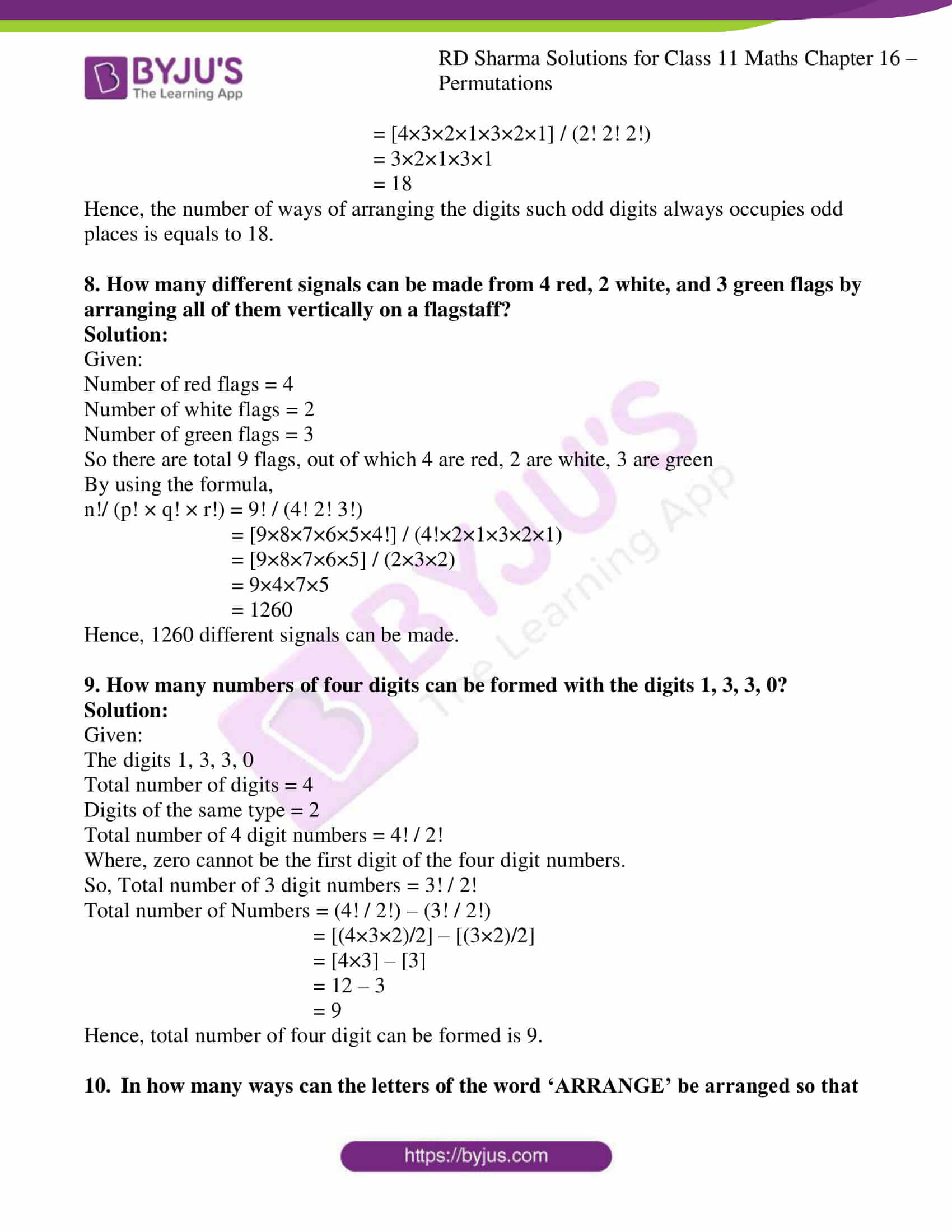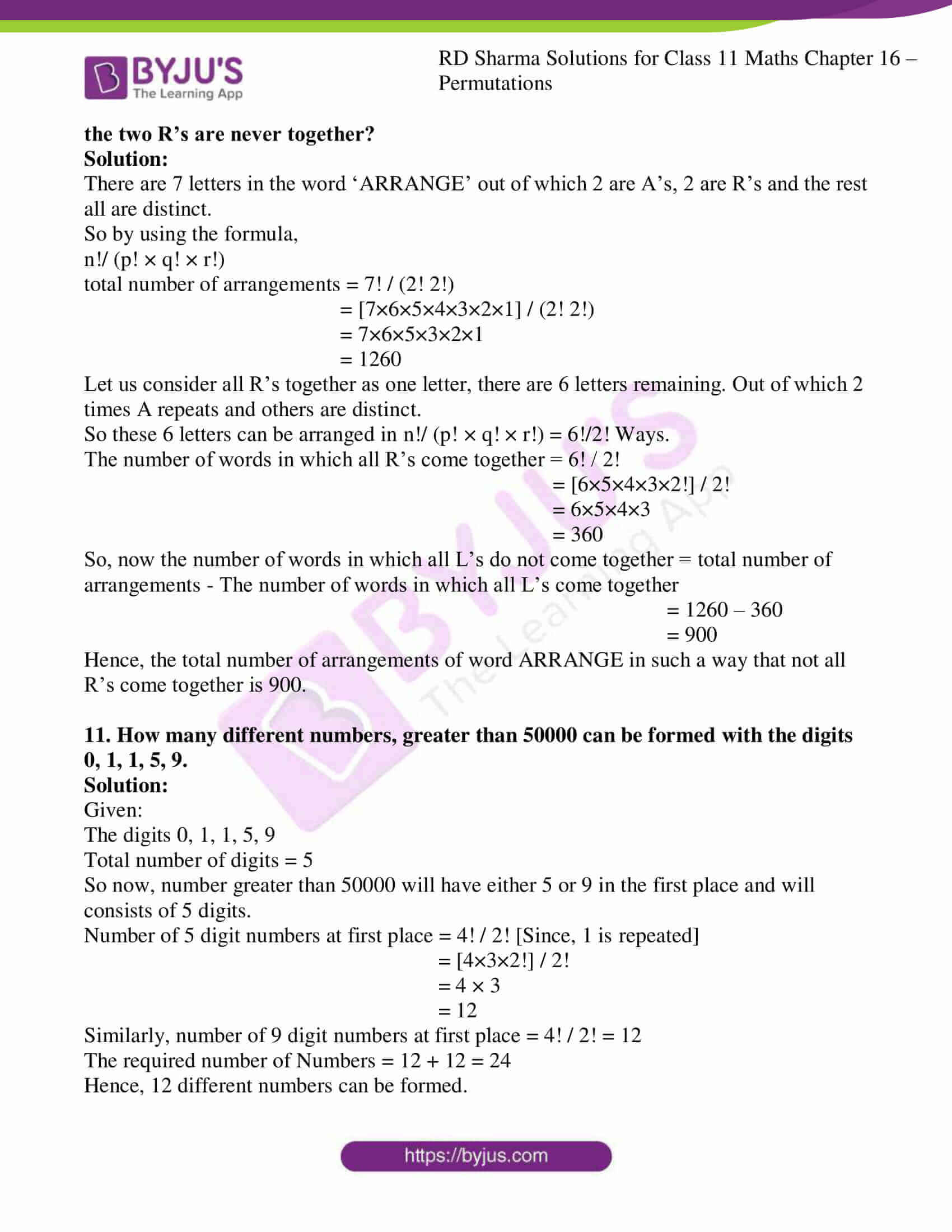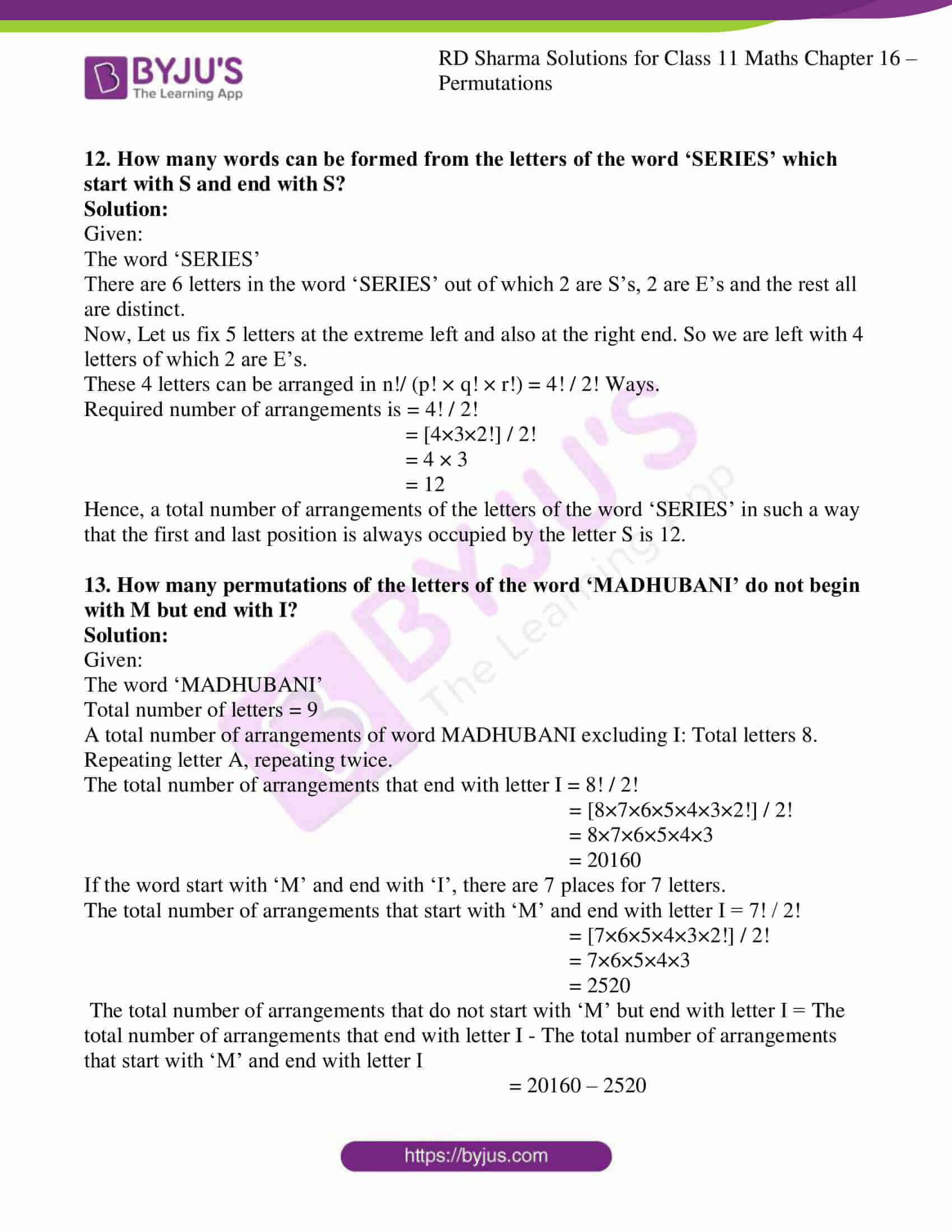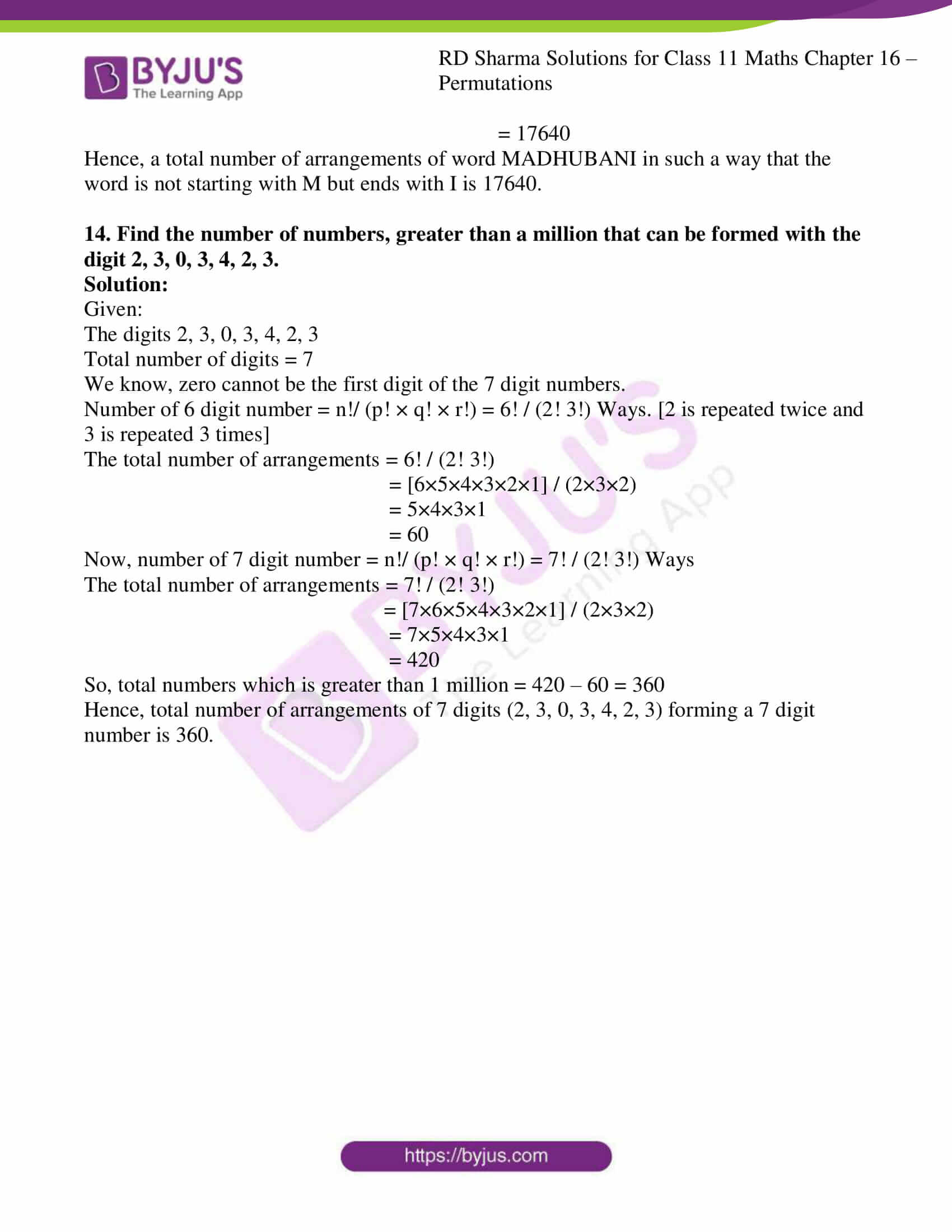### Access answers to RD Sharma Solutions for Class 11 Maths Exercise 16.5 Chapter 16 – Permutations

1. Find the number of words formed by permuting all the letters of the following words :
(i) INDEPENDENCE

(ii) INTERMEDIATE

(iii) ARRANGE

(iv) INDIA

(v) PAKISTAN

(vi) RUSSIA

(vii) SERIES

(viii) EXERCISES

(ix) CONSTANTINOPLE

Solution:

(i) INDEPENDENCE

There are 12 letters in the word ‘INDEPENDENCE’ out of which 2 are D’s, 3 are N’s, 4 are E’s and the rest all are distinct.

So by using the formula,

n!/ (p! × q! × r!)

total number of arrangements = 12! / (2! 3! 4!)

= [12×11×10×9×8×7×6×5×4×3×2×1] / (2! 3! 4!)

= [12×11×10×9×8×7×6×5] / (2×1×3×2×1)

= 11×10×9×8×7×6×5

= 1663200

(ii) INTERMEDIATE

There are 12 letters in the word ‘INTERMEDIATE’ out of which 2 are I’s, 2 are T’s, 3 are E’s and the rest all are distinct.

So by using the formula,

n!/ (p! × q! × r!)

total number of arrangements = 12! / (2! 2! 3!)

= [12×11×10×9×8×7×6×5×4×3×2×1] / (2! 2! 3!)

= [12×11×10×9×8×7×6×5×3×2×1] / (3!)

= 12×11×10×9×8×7×6×5

= 19958400

(iii) ARRANGE

There are 7 letters in the word ‘ARRANGE’ out of which 2 are A’s, 2 are R’s and the rest all are distinct.

So by using the formula,

n!/ (p! × q! × r!)

total number of arrangements = 7! / (2! 2!)

= [7×6×5×4×3×2×1] / (2! 2!)

= 7×6×5×3×2×1

= 1260

(iv) INDIA

There are 5 letters in the word ‘INDIA’ out of which 2 are I’s and the rest all are distinct.

So by using the formula,

n!/ (p! × q! × r!)

total number of arrangements = 5! / (2!)

= [5×4×3×2×1] / 2!

= 5×4×3

= 60

(v) PAKISTAN

There are 8 letters in the word ‘PAKISTAN’ out of which 2 are A’s and the rest all are distinct.

So by using the formula,

n!/ (p! × q! × r!)

total number of arrangements = 8! / (2!)

= [8×7×6×5×4×3×2×1] / 2!

= 8×7×6×5×4×3

= 20160

(vi) RUSSIA

There are 6 letters in the word ‘RUSSIA’ out of which 2 are S’s and the rest all are distinct.

So by using the formula,

n!/ (p! × q! × r!)

total number of arrangements = 6! / (2!)

= [6×5×4×3×2×1] / 2!

= 6×5×4×3

= 360

(vii) SERIES

There are 6 letters in the word ‘SERIES’ out of which 2 are S’s, 2 are E’s and the rest all are distinct.

So by using the formula,

n!/ (p! × q! × r!)

total number of arrangements = 6! / (2! 2!)

= [6×5×4×3×2×1] / (2! 2!)

= 6×5×3×2×1

= 180

(viii) EXERCISES

There are 9 letters in the word ‘EXERCISES’ out of which 3 are E’s, 2 are S’s and the rest all are distinct.

So by using the formula,

n!/ (p! × q! × r!)

total number of arrangements = 9! / (3! 2!)

= [9×8×7×6×5×4×3×2×1] / (3! 2!)

= [9×8×7×6×5×4×3×2×1] / (3×2×1×2×1)

= 9×8×7×5×4×3×1

= 30240

(ix) CONSTANTINOPLE

There are 14 letters in the word ‘CONSTANTINOPLE’ out of which 2 are O’s, 3 are N’s, 2 are T’s and the rest all are distinct.

So by using the formula,

n!/ (p! × q! × r!)

total number of arrangements = 14! / (2! 3! 2!)

= 14!/ (2×1×3×2×1×2×1)

= 14! / 24

2. In how many ways can the letters of the word ‘ALGEBRA’ be arranged without changing the relative order of the vowels and consonants?

Solution:

Given:

The word ‘ALGEBRA’

There are 4 consonants in the word ‘ALGEBRA’

The number of ways to arrange these consonants is 4P4 = 4!

There are 3 vowels in the word ‘ALGEBRA’ of which, 2 are A’s

So vowels can be arranged in n!/ (p! × q! × r!) = 3! / 2! Ways

Hence, the required number of arrangements = 4! × (3! / 2!)

= [4×3×2×1×3×2×1] / (2×1)

= 4×3×2×1×3 = 72

3. How many words can be formed with the letters of the word ‘UNIVERSITY,’ the vowels remaining together?

Solution:

Given:

The word ‘UNIVERSITY’

There are 10 letters in the word ‘UNIVERSITY’ out of which 2 are I’s

There are 4 vowels in the word ‘UNIVERSITY’ out of which 2 are I’s

So these vowels can be put together in n!/ (p! × q! × r!) = 4! / 2! Ways

Let us consider these 4 vowels as one letter, remaining 7 letters can be arranged in 7! Ways.

Hence, the required number of arrangements = (4! / 2!) × 7!

= (4×3×2×1×7×6×5×4×3×2×1) / (2×1)

= 4×3×2×1×7×6×5×4×3

= 60480

4. Find the total number of arrangements of the letters in the expression a3 b2 c4 when written at full length.

Solution:

There are 9 (i.e powers 3 + 2 + 4 = 9) objects in the expression a3 b2 c4 and there are 3 a’s, 2 b’s, 4 c’s

So by using the formula,

n!/ (p! × q! × r!)

total number of arrangements = 9! / (3! 2! 4!)

= [9×8×7×6×5×4×3×2×1] / (3×2×1×2×1×4×3×2×1)

= 7×6×5×3×2×1

= 1260

5. How many words can be formed with the letters of the word ‘PARALLEL’ so that all L’s do not come together?

Solution:

Given:

The word ‘PARALLEL’

There are 8 letters in the word ‘PARALLEL’ out of which 2 are A’s, 3 are L’s and the rest all are distinct.

So by using the formula,

n!/ (p! × q! × r!)

total number of arrangements = 8! / (2! 3!)

= [8×7×6×5×4×3×2×1] / (2×1×3×2×1)

= 8×7×5×4×3×1

= 3360

Now, let us consider all L’s together as one letter, so we have 6 letters out of which A repeats 2 times and others are distinct.

These 6 letters can be arranged in 6! / 2! Ways.

The number of words in which all L’s come together = 6! / 2!

= [6×5×4×3×2×1] / (2×1)

= 6×5×4×3

= 360

So, now the number of words in which all L’s do not come together = total number of arrangements – The number of words in which all L’s come together

= 3360 – 360 = 3000

6. How many words can be formed by arranging the letters of the word ‘MUMBAI’ so that all M’s come together?

Solution:

Given:

The word ‘MUMBAI’

There are 6 letters in the word ‘MUMBAI’ out of which 2 are M’s and the rest all are distinct.

So let us consider both M’s together as one letter, the remaining 5 letters can be arranged in 5! Ways.

Total number of arrangements = 5!

= 5×4×3×2×1

= 120

Hence, a total number of words formed during the arrangement of letters of word MUMBAI such that all M’s remains together equals to 120.

7. How many numbers can be formed with the digits 1, 2, 3, 4, 3, 2, 1 so that the odd digits always occupy the odd places?

Solution:

Given:

The digits 1, 2, 3, 4, 3, 2, 1

The total number of digits are 7.

There are 4 odd digits 1,1,3,3 and 4 odd places (1,3,5,7)

So, the odd digits can be arranged in odd places in n!/ (p! × q! × r!) = 4!/(2! 2!) ways.

The remaining even digits 2,2,4 can be arranged in 3 even places in n!/ (p! × q! × r!) = 3!/2! Ways.

Hence, the total number of digits = 4!/(2! 2!) × 3!/2!

= [4×3×2×1×3×2×1] / (2! 2! 2!)

= 3×2×1×3×1

= 18

Hence, the number of ways of arranging the digits such odd digits always occupies odd places is equals to 18.

8. How many different signals can be made from 4 red, 2 white, and 3 green flags by arranging all of them vertically on a flagstaff?

Solution:

Given:

Number of red flags = 4

Number of white flags = 2

Number of green flags = 3

So there are total 9 flags, out of which 4 are red, 2 are white, 3 are green

By using the formula,

n!/ (p! × q! × r!) = 9! / (4! 2! 3!)

= [9×8×7×6×5×4!] / (4!×2×1×3×2×1)

= [9×8×7×6×5] / (2×3×2)

= 9×4×7×5

= 1260

Hence, 1260 different signals can be made.

9. How many numbers of four digits can be formed with the digits 1, 3, 3, 0?

Solution:

Given:

The digits 1, 3, 3, 0

Total number of digits = 4

Digits of the same type = 2

Total number of 4 digit numbers = 4! / 2!

Where, zero cannot be the first digit of the four digit numbers.

So, Total number of 3 digit numbers = 3! / 2!

Total number of Numbers = (4! / 2!) – (3! / 2!)

= [(4×3×2)/2] – [(3×2)/2]

= [4×3] – 

= 12 – 3

= 9

Hence, total number of four digit can be formed is 9.

10. In how many ways can the letters of the word ‘ARRANGE’ be arranged so that the two R’s are never together?

Solution:

There are 7 letters in the word ‘ARRANGE’ out of which 2 are A’s, 2 are R’s and the rest all are distinct.

So by using the formula,

n!/ (p! × q! × r!)

total number of arrangements = 7! / (2! 2!)

= [7×6×5×4×3×2×1] / (2! 2!)

= 7×6×5×3×2×1

= 1260

Let us consider all R’s together as one letter, there are 6 letters remaining. Out of which 2 times A repeats and others are distinct.

So these 6 letters can be arranged in n!/ (p! × q! × r!) = 6!/2! Ways.

The number of words in which all R’s come together = 6! / 2!

= [6×5×4×3×2!] / 2!

= 6×5×4×3

= 360

So, now the number of words in which all L’s do not come together = total number of arrangements – The number of words in which all L’s come together

= 1260 – 360

= 900

Hence, the total number of arrangements of word ARRANGE in such a way that not all R’s come together is 900.

11. How many different numbers, greater than 50000 can be formed with the digits 0, 1, 1, 5, 9.

Solution:

Given:

The digits 0, 1, 1, 5, 9

Total number of digits = 5

So now, number greater than 50000 will have either 5 or 9 in the first place and will consists of 5 digits.

Number of 5 digit numbers at first place = 4! / 2! [Since, 1 is repeated]

= [4×3×2!] / 2!

= 4 × 3

= 12

Similarly, number of 9 digit numbers at first place = 4! / 2! = 12

The required number of Numbers = 12 + 12 = 24

Hence, 12 different numbers can be formed.

12. How many words can be formed from the letters of the word ‘SERIES’ which start with S and end with S?

Solution:

Given:

The word ‘SERIES’

There are 6 letters in the word ‘SERIES’ out of which 2 are S’s, 2 are E’s and the rest all are distinct.

Now, Let us fix 5 letters at the extreme left and also at the right end. So we are left with 4 letters of which 2 are E’s.

These 4 letters can be arranged in n!/ (p! × q! × r!) = 4! / 2! Ways.

Required number of arrangements is = 4! / 2!

= [4×3×2!] / 2!

= 4 × 3

= 12

Hence, a total number of arrangements of the letters of the word ‘SERIES’ in such a way that the first and last position is always occupied by the letter S is 12.

13. How many permutations of the letters of the word ‘MADHUBANI’ do not begin with M but end with I?

Solution:

Given:

Total number of letters = 9

A total number of arrangements of word MADHUBANI excluding I: Total letters 8. Repeating letter A, repeating twice.

The total number of arrangements that end with letter I = 8! / 2!

= [8×7×6×5×4×3×2!] / 2!

= 8×7×6×5×4×3

= 20160

If the word start with ‘M’ and end with ‘I’, there are 7 places for 7 letters.

The total number of arrangements that start with ‘M’ and end with letter I = 7! / 2!

= [7×6×5×4×3×2!] / 2!

= 7×6×5×4×3

= 2520

The total number of arrangements that do not start with ‘M’ but end with letter I = The total number of arrangements that end with letter I – The total number of arrangements that start with ‘M’ and end with letter I

= 20160 – 2520

= 17640

Hence, a total number of arrangements of word MADHUBANI in such a way that the word is not starting with M but ends with I is 17640.

14. Find the number of numbers, greater than a million that can be formed with the digit 2, 3, 0, 3, 4, 2, 3.

Solution:

Given:

The digits 2, 3, 0, 3, 4, 2, 3

Total number of digits = 7

We know, zero cannot be the first digit of the 7 digit numbers.

Number of 6 digit number = n!/ (p! × q! × r!) = 6! / (2! 3!) Ways. [2 is repeated twice and 3 is repeated 3 times]

The total number of arrangements = 6! / (2! 3!)

= [6×5×4×3×2×1] / (2×3×2)

= 5×4×3×1

= 60

Now, number of 7 digit number = n!/ (p! × q! × r!) = 7! / (2! 3!) Ways

The total number of arrangements = 7! / (2! 3!)

= [7×6×5×4×3×2×1] / (2×3×2)

= 7×5×4×3×1

= 420

So, total numbers which is greater than 1 million = 420 – 60 = 360

Hence, total number of arrangements of 7 digits (2, 3, 0, 3, 4, 2, 3) forming a 7 digit number is 360.